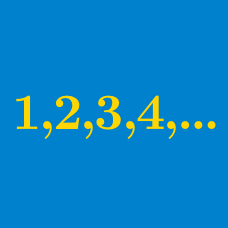Algebra

# Arithmetic Progression Properties

Which of these sequences are arithmetic progressions?

$\text{A: }\{16, 12, 8, 4\}$ $\text{B: }\{8, 3, -2, -7\}$

Which of these sequences are arithmetic progressions?

$\text{A: }\left\{\frac{3}{2},\frac{5}{2},\frac{7}{2}\right\}$ $\text{B: }\left\{\frac{5}{3},3,\frac{13}{3}\right\}$

Which of these sequences are arithmetic progressions?

$\text{A: }\{6,4,2,0,-2,-4,-6\}$ $\text{B: }\{6,4,2,0,2,4,6\}$

Which of these sequences are arithmetic progressions?

$\text{A: }\{-1,3,-5,7,-9\}$ $\text{B: }\{-9,-5,-1,3,7\}$

Which of these sequences are arithmetic progressions?

$\text{A: }\{-10,-5,5,10,15\}$ $\text{B: }\{-10,-5,0,5,10\}$

×